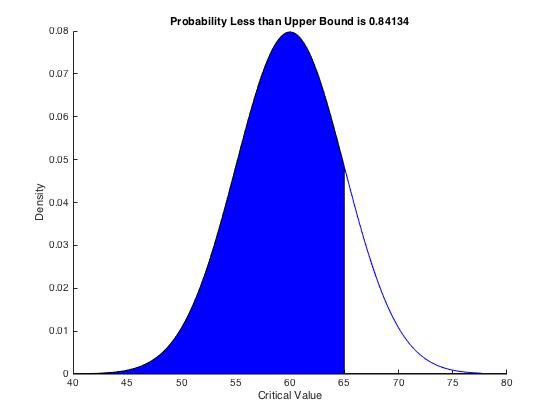# An Undiscovered MATLAB Function

Regular readers here will know that I love using MATLAB for some things but earlier this week I discovered a function that I hadn’t used before and is surprisingly useful.

I wanted to produce some diagrams showing portions of a normal distribution shaded and had tried to do it in Geogebra but wasn’t quite happy with the results. I then decided to try R but for some reason (probably to do with X-Windows or Quartz) the graphics output on the R installation on my Mac wasn’t working properly so I fired up MATLAB. My original intention was to plot the normal distribution function I was interested in, then plot the vertical lines at the limits and then shade the region. However, I discovered that in the “Statistics and Machine Learning Toolbox” there was a function called $$\texttt{normspec}$$.

The syntax for this function is described in detail in the documentation but essentially this function can be used to shade a region under a given normal distribution. The syntax is $$\texttt{p = normspec(specs,mu,sigma)}$$ and this plots the normal distribution with mean $$\texttt{mu}$$ and standard deviation $$\texttt{sigma}$$. It then shades the portion inside the specification limits given by the two element vector $$\texttt{specs}$$ – you can use $$\texttt{-Inf}$$ or $$\texttt{Inf}$$ if there is no lower or upper limit respectively.

For example, the command $$\texttt{p = normspec([-Inf,65],65,5)}$$ produces the plot shown below:I’d encourage you to go and try it out if you have a MATLAB licence.